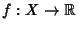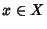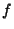## Birkhoff's Ergodic Theorem

Letbe an ergodic Endomorphism of the Probability Spaceand letbe a real-valued Measurable Function. Then for Almost Every, we have(1)

as. To illustrate this, taketo be the characteristic function of some Subsetofso that(2)

The left-hand side of (1) just says how often the orbit of(that is, the points,,, ...) lies in, and the right-hand side is just the Measure of. Thus, for an ergodic Endomorphism, space-averages = time-averages almost everywhere.'' Moreover, ifis continuous and uniquely ergodic with Borel Probability Measureandis continuous, then we can replace the Almost Everywhere convergence in (1) to everywhere.